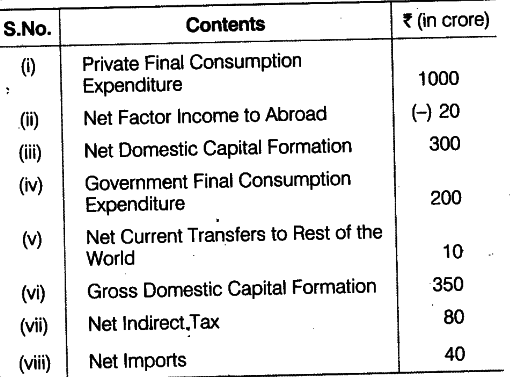# Calculate National Income

Calculate (a) National Income and (b) Gross National Disposable Income.(a) National Income (NN\${{P}{FC}}\$) = Private Final Consumption Expenditure + Government Final Consumption Expenditure + Net Domestic Capital Formation - Net Imports - Net Indirect Tax - Net Factor Income to Abroad
=1000 +200 + 300 - 40 - 80 - (- 20)
=1520—120 = Rs. 1400 crore
(b) Gross National Disposable Income = NN\${{P}
{FC}}\$ + Net Indirect Tax + Depreciation - Net Current Transfers to Rest of the World = 1400 + 80 + 50 -10 = Rs. 1520 crore